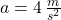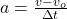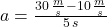## A racecar driver on a flat racetrack steps on the gas, changing her velocity from 10 m/sec to 30 m/sec in 5 seconds. What is the acceleratio

Question

A racecar driver on a flat racetrack steps on the gas, changing her velocity from 10 m/sec to 30 m/sec in 5 seconds. What is the acceleration of the racecar during that time frame?

in progress 0
2 months 2021-07-30T22:03:57+00:00 2 Answers 3 views 0

4m/s2

Explanation:

The following data were obtained from the question:

U (initial velocity) = 10m/s

V (final velocity) = 30m/s

t (time) = 5secs

a (acceleration) =?

Acceleration is the rate of change of velocity with time. It is represented mathematically as:

a = (V – U)/t

Now, with this equation i.e

a = (V – U)/t, we can calculate the acceleration of the race car as follow:

a = (V – U)/t

a = (30 – 10)/5

a = 20/5

a = 4m/s2

Therefore, the acceleration of the race car is 4m/s2